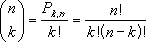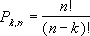## Excel Office

Excel How Tos, Tutorials, Tips & Tricks, Shortcuts

# COMBIN function: Description, Usage, Syntax, Examples and Explanation

## What is COMBIN function in Excel?

COMBIN function is one of the Math and Trig functions in Microsoft Excel that returns the number of combinations for a given number of items. Use COMBIN to determine the total possible number of groups for a given number of items.

## Syntax of COMBIN function

COMBIN(number, number_chosen)

The COMBIN function syntax has the following arguments:

• Number: The number of items.
• Number_chosen: The number of items in each combination.

## COMBIN formula explanation

• Numeric arguments are truncated to integers.
• If either argument is nonnumeric, COMBIN returns the #VALUE! error value.
• If number < 0, number_chosen < 0, or number < number_chosen, COMBIN returns the #NUM! error value.
• A combination is any set or subset of items, regardless of their internal order. Combinations are distinct from permutations, for which the internal order is significant.
• The number of combinations is as follows, where number = n and number_chosen = k:where:## Example of COMBIN function

Steps to follow:

1. Open a new Excel worksheet.

2. Copy data in the following table below and paste it in cell A1

Note: For formulas to show results, select them, press F2 key on your keyboard and then press Enter.

You can adjust the column widths to see all the data, if need be.

 Formula Description Result =COMBIN(8,2) Possible two-person teams that can be formed from 8 candidates. 28